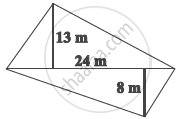Share

# The Diagonal of a Quadrilateral Shaped Field is 24 M and the Perpendiculars Dropped on It from the Remaining Opposite Vertices Are 8 M and 13 M. Find the Area of the Field. - CBSE Class 8 - Mathematics

#### Question

The diagonal of a quadrilateral shaped field is 24 m and the perpendiculars dropped on it from the remaining opposite vertices are 8 m and 13 m. Find the area of the field.#### Solution

It is given that,

Length of the diagonal, = 24 m

Length of the perpendiculars, h1 and h2, from the opposite vertices to the diagonal are h1 = 8 m and h2 = 13 m

Area of the quadrilateral = 1/2 d(h_1 + h_2)

= 1/2 (24m) x (13m + 8cm)

= 1/2 (24 m)(21 m)

= 252 m^2

Thus, the area of the field is 252 m2.

Is there an error in this question or solution?

#### APPEARS IN

NCERT Solution for Mathematics Textbook for Class 8 (2018 to Current)
Chapter 11: Mensuration
Ex. 11.20 | Q: 4 | Page no. 178
Solution The Diagonal of a Quadrilateral Shaped Field is 24 M and the Perpendiculars Dropped on It from the Remaining Opposite Vertices Are 8 M and 13 M. Find the Area of the Field. Concept: Area of a General Quadrilateral.
S